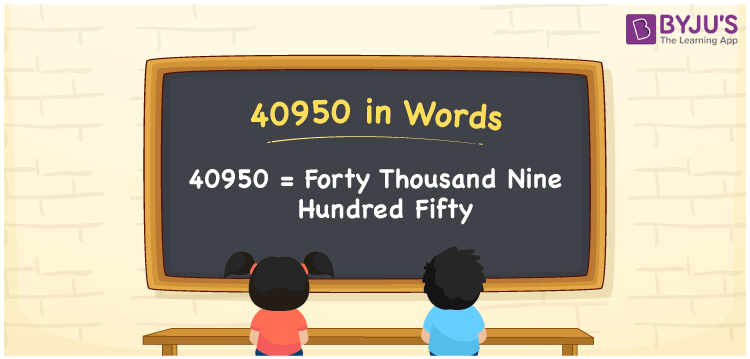# 40950 in words

40950 in words is written as Forty Thousand Nine Hundred and Fifty. In 40950, 4 has a place value of ten thousand, 9 is in the place value of hundred and 5 is in the place value of ten. The article on Place Value gives more information. The number 40950 is used in expressions that relate to money, distance, social media views, and many more. For example, “The overhead water tank has a capacity of Forty Thousand Nine Hundred and Fifty liters”.

 40950 in words Forty Thousand Nine Hundred and Fifty Forty Thousand Nine Hundred and Fifty in Numbers 40950

## 40950 in English Words## How to Write 40950 in Words?

We can convert 40950 to words using a place value chart. The number 40950 has 5 digits, so let’s make a chart that shows the place value up to 5 digits.

 Ten thousand Thousands Hundreds Tens Ones 4 0 9 5 0

Thus, we can write the expanded form as:

4 × Ten thousand + 0 × Thousand + 9 × Hundred + 5 × Ten + 0 × One

= 4 × 10000 + 0 × 1000 + 9 × 100 + 5 × 10 + 0 × 1

= 40950.

= Forty Thousand Nine Hundred and Fifty.

40950 is the natural number that is succeeded by 40949 and preceded by 40951.

40950 in words – Forty Thousand Nine Hundred and Fifty.

Is 40950 an odd number? – No.

Is 40950 an even number? – Yes.

Is 40950 a perfect square number? – No.

Is 40950 a perfect cube number? – No.

Is 40950 a prime number? – No.

Is 40950 a composite number? – Yes.

## Solved Example

1. Write the number 40950 in expanded form

Solution: 4 x 10000 + 0 x 1000 + 9 x 100 + 5 x 10 + 0 x 1

We can write 40950 = 40000 + 0000 + 900 + 50 + 0

= 4 x 10000 + 0 x 1000 + 9 x 100 + 5 x 10 + 0 x 1.

## Frequently Asked Questions on 40950 in words

Q1

### How to write the number 40950 in words?

40950 in words is written as Forty Thousand Nine Hundred and Fifty.
Q2

### Is 40950 a prime number?

No. 40950 is not a prime number.
Q3

### Is 40950 divisible by 10?

Yes. 40950 is divisible by 10.